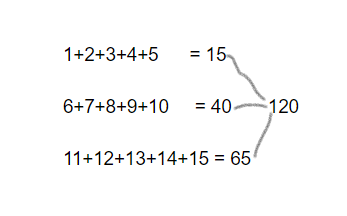2 views

I am new to Spark and Scala. I was confused about the way reduceByKey function works in Spark. Suppose we have the following code:

val lines = sc.textFile("data.txt")
val pairs = lines.map(s => (s, 1))
val counts = pairs.reduceByKey((a, b) => a + b)

The map function is clear: s is the key and it points to the line from data.txt and 1 is the value.

However, I didn't get how the reduceByKey works internally?

+1 vote
by (32.3k points)

When a reduceByKey function is applied on a Spark RDD, it merges the values for each key by using an associative reduce function.

Associative functions helps us in doing parallel computation. Here we break an original collection into pieces and then apply the associative function, that is further accumulated as a total.

Also, Associativity allows us to use that same function in sequence and in parallel.

This property is used by reduceByKey transformation in order to compute a result out of an RDD.

Consider the following example:

// Here, collection of the form ("key",1),("key,2),...,("key",15) splits among 3 partitions

val rdd =sparkContext.parallelize(( (1 to 15).map(x=>("key",x))), 3)

rdd.reduceByKey(_ + _)

rdd.collect()

> Array[(String, Int)] = Array((key,))In spark, data is distributed into partitions. For the next illustration, (3) partitions are to the left, enclosed in thin lines. First, we apply the function locally to each partition, sequentially in the partition, but we run all 3 partitions in parallel. After that, the result of each local computation is aggregated by applying the same function again and eventually the result is produced.